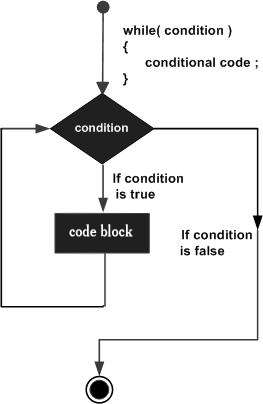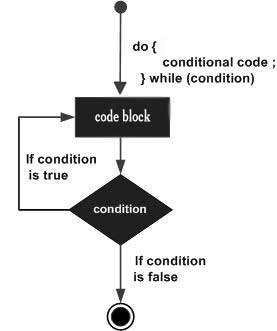# Difference Between while and do-while Loop

In this post, we will understand the difference between the ‘while’ loop and the ‘do-while’ loop.

## while condition

• The controlling condition here appears at the beginning of the loop.

• The iterations do not occur if the condition at the first iteration results in False.

• It is also known as an entry-controlled loop

• There is no condition at the end of the loop.

• It doesn’t need to execute at least one.

## Example

while ( condition){
statements;
//body of loop
}

Following is the flowchart of while loop −## do-while condition

• The controlling condition is present at the end of the loop.

• The condition is executed at least once even if the condition computes to false during the first iteration.

• It is also known as an exit-controlled loop

• There is a condition at the end of the loop.

## Example

do {
statements;
// body of loop.
}
while( Condition );

Following is the flowchart of do-while loop −